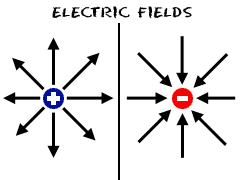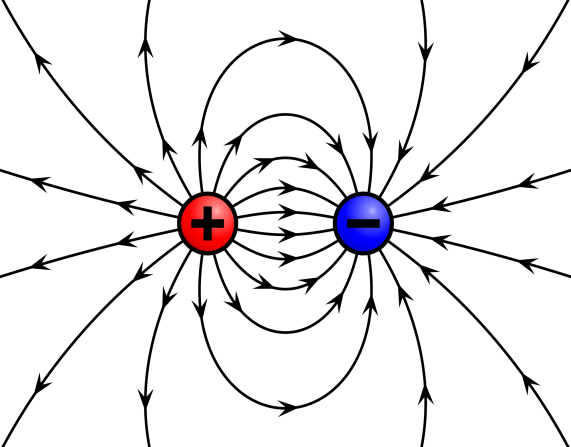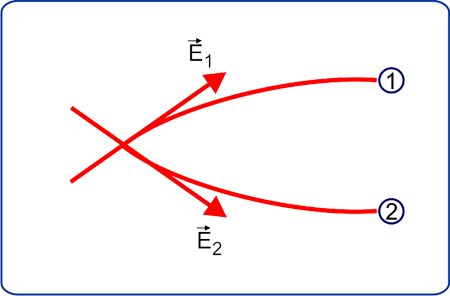Electric Field Lines for JEE

Electrostatics for IIT JEE - Electric Field Lines Characteristics

In Physics every field is inter-related. If we point out one thing, then we must know about the details of other things which have some connection with others. Today we are going to discuss the electric field lines. For this, we must have some knowledge about the electric field. Thus, first, we will discuss “Electric Field”.

Electric field

A field can be defined as a movable variable like (x, y, z) φ. It is kind of omnipresent because it has its existence everywhere. It has a general tendency to change from one place to another place. Now if we talk about mathematical terms, we can say that a field is a function of 3 types of coordinates which are x, y and z. Moreover if fields are time-dependent, then these fields are a function of the time φ(x, y, z, and t). So now we can deduce that some physical fields are scalars, in other words, we can understand by this simple line i.e., the field φ (x, y, and z) is a scalar at every point (x, y, and z). On the other hand, the examples of vector fields are the magnetic field B (x, y, and z) and the electric field E (x, y, and z). Since an electric field is a vector, thus electric field can be represented with arrows which have been drawn at various points around an object with charge.

Electric Field lines

Now the question arises that what will be the best way to conceptualize about how a vector field (E (x, y, and z)) changes its magnitude and direction from one place to another and how to show it? The answer is by drawing “Field Lines”. The direction of the vector field is being the line’s direction at that point where a field line along at every point. In very simple terms you can say that Electric field lines are those curves which display the direction of an electric field when a tangent is drawn at its point. If there is any need to describe the overall intensity of the field, an electric field is used in the pictorial form. You can call these pictorial representations as electric field lines. Lines of force or electric field lines have been drawn below to present some examples:In the above figure, there are two sides wherein the left-hand side shows field lines around a positive object on the other hand in the right-hand side it shows field lines around a negative object. These lines, which are drawn to show the directions, are showing the direction of force owing to the electric field because it acts on the positive charge. It is the general tendency of lines that they get closer to each other when you get closer to the charge. It explains one thing; the more you go closer to the main source, the more electric field gets stronger. On the contrary, if you place yourself further out, the field becomes weaker and field lines are no denser than before.In the above image, we can see what happens when you place two opposite charge close enough to each other so that the interactions between their field lines can happen easily. Now we can conclude after seeing the above images that the arrows are going in exactly the same direction one could expect from a positive charge to move i.e., from the positive charge to the negative charge. The tangent is drawn to the field line at any point in the direction of the field at that point.

Important features of Electric field lines-

Now we take some examples to understand these properties. There are numerous features of Electric Field Lines which need to be discussed. Now we will discuss them in details:

1. The foremost features of the electric field lines are that they never cross or intersect each other. Somehow if two electric field lines cross each other than one situation will occur where two vectors will be depicting two directions of the same electric field and this can’t be possible.
2. It is the general tendency of the electric field lines that they start on the positive charge and end on the negative charge and that are why they do not form any closed curve.
3. Electric field lines always end at infinity if it’s a case of a single charge.
4. The magnitude of the charge is always proportional to the number of electric field lines which are leaving positive charge or entering the negative charge.
5. If electric field lines are smooth and continuous it means that they are in a charge-free region.
6. The electric field lines are always parallel, systematically spaced and straight showing that they are in a uniform electric field.
7. If we talk about the flow of the electric field lines, they always flow from the higher potential to the lower potential.
8. Electric field lines lose their existence if the electric field of space in a given region is zero.
9. The direction of the electric field is given by the tangent to a line at any point. Moreover, if a positive charge is free to move then this would be the path on which it will tend to move.
10. When magnetic and electric both fields are present and changed with time then looping electric field lines appears in electrodynamics. However, in electrostatics, the electric field lines do not form any closed loops.

Some important examples-

Example 1- Know about Electric field lines as Invisible reality

First, we must know why was it necessary to discover the concept of the electric field? What was the need to know about the concept of Electric field?

The concept of Electric field emerged because it was an attempt by scientists to explain the action at a distance that appears between charged objects. The first person who introduced the concept of electric field was 19th-century physicist Michael Faraday. It was Faraday who told that the pattern of lines identifying the electric field represents an invisible reality. Faraday chose to apply the concept of a field to propose that a massive object (in case of the gravitational field) or charge object affects the space around it over had a thought about one charge affecting another charge. The field which has been established in that space affects another object which enters in that space. A charge which is seen to interact with an electric field always opposes another charge. According to Faraday if you want to understand action-at-a-distance you must have the understanding of the power of charge-field-charge. The electric field of a charged object is sent into space, reaching from the “puller to the pullee”.

An intricate web of influence in the space surrounding it is being created by each charge or configuration of charges. The effect is real if lines are invisible. Now we can say that if you are practising the exercise of construction of electric field lines around the configuration of charges or charges you are not doing anything else. In fact, you are simply drawing the curvy lines. Somewhat, you are just elaborating the electrified web of space which will repel and draw other charges that take an entry in it.

Example 2- How come is it possible that Electric field lines never intersect each other?

In a system of charges, the nature of electric field lines is told by Electric field lines. Moreover, electric field lines disclose about the direction of electric field strength in a region of space at a point. There must be exclusively two electric fields with different directions if the electric field lines intersect. However, it is not possible since the solitary location has only one direction and
magnitude of the electric field associated with it. This below figure can easily explain the above term:(Suppose lines are intersecting, there must be two directions of the same electric field though it cannot be possible)

Applicability of Electric field lines-

Some people say that it won’t be good enough to think “Electric Field” as lines. Rather you can have a thought about this concept as a “Cloud”. Lines basically denote that E field is zero between the lines and exists on the line. The Electric Field lines are not detached like this. Moreover, Electric field is very uninterrupted and smooth. You can call them a vector because of the points in the same direction where they ever exist. It simply implies that once someone follows the direction of the vector it can be understood that the line is really what is formed. If we talk about the fundamental use of these lines, we get to know that the use of the lines is totally dependent on the interaction of the field with the sensors and other devices. The alignment of the antenna or sensor with lines is the most important thing to be noticed because in the absence of this feature, the antenna or the sensor cannot detect the electric field. The lines also guide a way to envisage the direction of the electric field. This whole scenario makes everyone capable to understand the procedure about how one device works. Anyone can use these lines as a visualization tool.